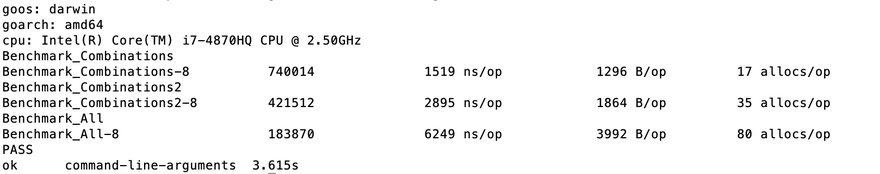There are various ways to implement the Go language to generate a Slice with a specified number of combinations, here are two with better performance.The first two are specified to take the combination length, the first one is faster, the second one has simple code and slightly worse performance, and the third one is a full combination.

The algorithm of the first one is implemented by Python’s itertools algorithm and is really fast.

 `````` 1 2 3 4 5 6 7 8 9 10 11 12 13 14 15 16 17 18 19 20 21 22 23 24 25 26 27 28 29 30 31 32 33 34 35 36 37 38 39 40 41 42 43 44 `````` ``````func Combinations(iterable []string, r int) (rt [][]string) { pool := iterable n := len(pool) if r > n { return } indices := make([]int, r) for i := range indices { indices[i] = i } result := make([]string, r) for i, el := range indices { result[i] = pool[el] } s2 := make([]string, r) copy(s2, result) rt = append(rt, s2) for { i := r - 1 for ; i >= 0 && indices[i] == i+n-r; i -= 1 { } if i < 0 { return } indices[i] += 1 for j := i + 1; j < r; j += 1 { indices[j] = indices[j-1] + 1 } for ; i < len(indices); i += 1 { result[i] = pool[indices[i]] } s2 = make([]string, r) copy(s2, result) rt = append(rt, s2) } } ``````

The second code is simple

 `````` 1 2 3 4 5 6 7 8 9 10 11 12 13 14 15 16 17 18 19 20 21 22 23 24 25 26 27 28 29 `````` ``````func Combinations2(set []string, n int) (subsets [][]string) { length := uint(len(set)) if n > len(set) { n = len(set) } // Go through all possible combinations of objects // from 1 (only first object in subset) to 2^length (all objects in subset) for subsetBits := 1; subsetBits < (1 << length); subsetBits++ { if n > 0 && bits.OnesCount(uint(subsetBits)) != n { continue } var subset []string for object := uint(0); object < length; object++ { // checks if object is contained in subset // by checking if bit 'object' is set in subsetBits if (subsetBits>>object)&1 == 1 { // add object to subset subset = append(subset, set[object]) } } // add subset to subsets subsets = append(subsets, subset) } return subsets } ``````

Incidentally, test the full combination

 `````` 1 2 3 4 5 6 7 8 9 10 11 12 13 14 15 16 17 18 19 20 21 `````` ``````func All(set []string) (subsets [][]string) { length := uint(len(set)) // Go through all possible combinations of objects // from 1 (only first object in subset) to 2^length (all objects in subset) for subsetBits := 1; subsetBits < (1 << length); subsetBits++ { var subset []string for object := uint(0); object < length; object++ { // checks if object is contained in subset // by checking if bit 'object' is set in subsetBits if (subsetBits>>object)&1 == 1 { // add object to subset subset = append(subset, set[object]) } } // add subset to subsets subsets = append(subsets, subset) } return subsets } ``````

## Test Results

 `````` 1 2 3 4 5 6 7 8 9 10 11 `````` ``````goos: darwin goarch: amd64 cpu: Intel(R) Core(TM) i7-4870HQ CPU @ 2.50GHz Benchmark_Combinations Benchmark_Combinations-8 740014 1519 ns/op 1296 B/op 17 allocs/op Benchmark_Combinations2 Benchmark_Combinations2-8 421512 2895 ns/op 1864 B/op 35 allocs/op Benchmark_All Benchmark_All-8 183870 6249 ns/op 3992 B/op 80 allocs/op PASS ok command-line-arguments 3.615s ``````